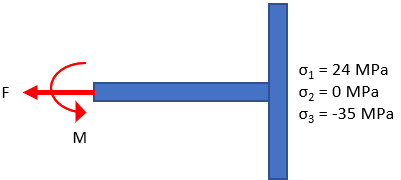## Max Shear Stress Theory

In the below figure, a ductile beam is in tension due to an applied load, F, which creates the three corresponding principal stresses. Does failure occur if the material’s yielding strength is 40 MPa?Hint
Max shear stress:
$$\tau_{max}=\frac{\sigma_1-\sigma_3}{2}$$$where $$\sigma$$ are the principal stresses. Hint 2 Yielding occurs when $$\tau_{max}\geq \frac{S_y}{2}$$$
where $$\tau_{max}$$ is the max shear stress, and $$S_y$$ is the yield strength.
For a ductile material in tension, the max shear stress theory predicts that yielding will occur whenever
$$\tau_{max}\geq \frac{S_y}{2}$$$where $$\tau_{max}$$ is the max shear stress, and $$S_y$$ is the yield strength. To find the max shear stress: $$\tau_{max}=\frac{\sigma_1-\sigma_3}{2}$$$
where $$\sigma$$ are the principal stresses. Thus,
$$\tau_{max}=\frac{24MPa-(-35MPa)}{2}=\frac{59MPa}{2}=29.5\:MPa$$$$$\frac{S_y}{2}=\frac{40MPa}{2}=20\:MPa$$$
Because $$29.5\:MPa\geq 20\:MPa$$ , failure does occur.
Failure occurs.